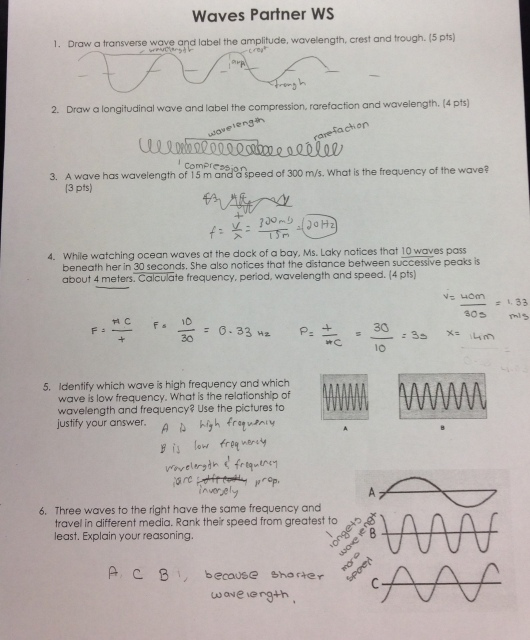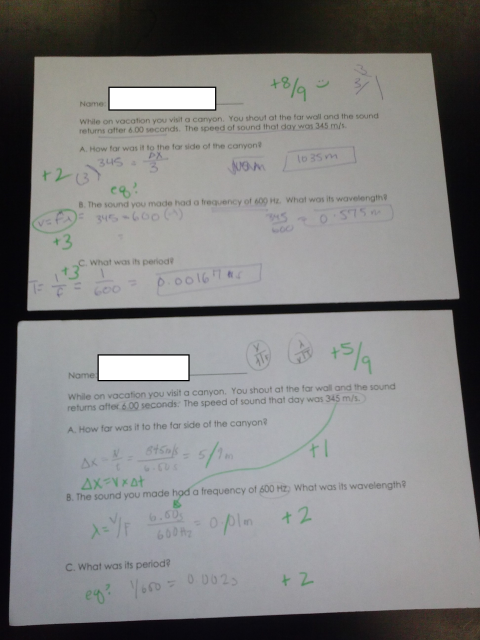# Using the Wave Equation to Solve Problems

4 teachers like this lesson
Print Lesson

## Objective

Students will be able to calculate the speed, frequency or wavelength of a wave if the other two quantities are known.

#### Big Idea

Students use the wave equation and explain how to solve wave equation problems to the class.

## Warm-Up Question: Wave Equation

10 minutes

The goal of this class is to give students practice using the wave equation. Students practice using computational thinking while solving various problems about types (SP5). They use multiple equations, requiring rearranging and selecting the right equation to use when solving for a specific variable. The wave equation relates the frequency, wavelength and speed (HS-PS4-1).

To start out class, I give my students a Wave Equation Warm Up. I do this so that I can give students a practice problem that requires them to solve for multiple quantities (speed, period and frequency) with the same given information. Students can see that even with a small amount of given information they can solve for many different variables. This is also a way to get the questions that my students have out before they work on the worksheet. I ask my students to spend about 3-4 minutes working on this problem individually. As students work, I walk around to see how they do. Students are able to find most of the answers prior to reviewing the answers. After a few minutes, I go through the answers on the whiteboard, asking my students to volunteer how they did each part of the problem. I emphasize that for period, frequency and wave speed there are multiple ways they can calculate them, depending on the order that they solved them.

## Wave Equation WS Work Time

25 minutes

After going through the warm-up questions I give the students about 25 minutes to complete the Wave Equation WS. Since they finished #1-5 on their own for homework, I ask them to compare answers with their group for the front page before they move onto working in the remaining problems on the back page. I have students work with their table groups so they can practice explaining the process to other students if they don't understand. As students are working, I walk around to make sure there are not any major questions.

## Review Wave Equation WS Problems

15 minutes

To go over the wave equation worksheet, I assign each table a problem or pair of problems to complete of the master copy of the Wave Equation Worksheet. Once each group is assigned problems, I ask one group member to come up to the front of the room to put their answers on the blank copy master copy for their class. When the students have completed their answers, Wave Equation WS Student Work, I show the answers on the document camera projected on the screen. I let students check their answers and then ask questions if they don't agree or understand how the group got an answer.

The reason that I review the problems this way is so that students can get all of the answers to the problems to check their work. As a formative assessment, I split these problems up so that each table has a set of problems so I can see how groups of students are doing on using the wave equation. This helps me see how well students are able to complete problems on wave speed.

## Partner Worksheet: All About Waves

15 minutes

After we have finished going over the wave equation worksheet, I have students chose one partner to work with for the Wave Partner WS. Before they move to find their partner, I tell them that this worksheet is a 6 problem summary of most of the things that we have learned so far this unit. Each partner writes for 3 problems, so I ask students to have one partner complete the odds and the other partner to complete the evens. I do this so each student gets practice writing for each part. I expect that students are checking over their partners work as they complete the worksheet.

After the instructions, I give students time to find their partners and I give them 10 minutes to complete the worksheet. As they work, I walk around to make sure students are on task and to answer any questions if both partners don't understand a problem. When the time is up, I show the answers up on the screen with two problems at a time. I show them two at a time because the first two questions are focused on labeling both types of waves, 3 & 4 are focused on using the wave equation and 5 & 6 are focused on qualitative relationships between wavelength and frequency. After each pair of problems, I ask students to give me a thumbs up if they got both problems correct. This gives me an idea of how my students did on each concept. One pair's work is shown in the image below.## Wave Equation Checkpoint

5 minutes

To end class, I have students complete the Wave Equation Checkpoint individually. There are three questions on this checkpoint that ask them to solve for different variables (speed, frequency, wavelength). They can solve for each of the quantities (speed, wavelength and period) without the answers to the other parts so I tell students if they cannot figure out A, they should move onto B or C because they can solve for the other two without finishing A. Since students have finished both the wave equation worksheet and the wave partner worksheet, I believe they are prepared to answer these questions. You can see student work shown below. Students turn this in as they finish to end class.